# Introducing Growth of Groups

March 14, 2017

The growth of a group is an aesthetically pleasing large-scale property that is borderline geometric and algorithmic.

I will give an overview on the research on growth by historical and intuitive examples.

## Introduction

I want to introduce you to the particularly beautiful group counting problem of how many elements can be spelled with $\leq n$ letters?

The growth function of a group is a large-scale geometric property that is connected to two out of three of Dehn’s decision problems: the isomorphism problem and the Word problem.

## Dehn’s Three Decision Problems

In 1911, the mathematician Max Dehn proposed three fundamental decision problems in group theory.

• The Word Problem: whether there is a procedure to determine whether two words in a group represent the same element.
• The Conjugacy Problem: whether there is a procedure to determine whether two words are conjugate to each other in a group.
• The Isomorphism Problem: whether there is procedure to determine whether two groups are isomorphic.

Dehn’s three questions are remarkable in that they precede by many years the formalization of “procedure” as “algorithm” by Church, Turing, et al. Source.

It is known by the work of Boone and Novikov in the 1954 that these three problems are undecidable.A problem is decidable if there exists a computable function giving an exact answer for any instance of a problem, undecidable when no computable function that can give an answer to every instance there exists. Source. The construction is not so direct as the Markov-Post construction and involves starting with free groups and performing a number of HNN-extensions and/or free products with amalgamation. Source Studying which groups have procedures and why is still an active area of research. I will be talking about growth, a property of groups which gives us some partial resolution to the Word problem and the isomorphism problem in terms of classifying groups.

## Gauss Circle Problem - Round Counting

Given a circle of radius $n$ centered at the origin of the lattice $\mathbb{Z}^2$, how does the number of points in the circle grow as $n \to \infty$?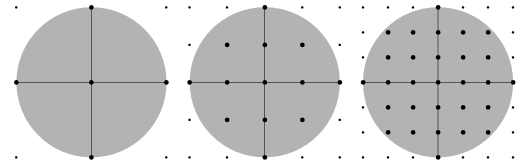The radius of the circle growing is equivalent to the elements of $\mathbb{Z}^2$ appearing closer and closer together.

Let $G(n)$ be the number of lattice points contained in the circle. A reasonable first guess is to count the lattice points with a Riemann sum. Indeed, the number of lattice points roughly corresponds to the number of unit squares contained in the circle. $n$ going to infinity is like “zooming out” on the lattice while keeping the size of the circle constant, and hence, as $n \to \infty$, the unit area becomes an infinitesimal area element.

Let $E(n)$ be the error term of our approximation. Then, a reasonable guess is that the area grows as

Remarking that the “fuzzy” part of our approximation comes at the boundary of the circle, we can arrive at Gauss’ conclusion in 1801 that the error term is at most linear,

which is also the optimal bound, as proven by Hardy and Landau.

## Changing the Norm - Pointy Counting (Optional)How the ball which encloses our vertices changes with the norm.

Our counting function $G(n)$ for the Gauss circle was implicitly defined as the intersection of $\mathbb{Z}^2$ and the closed Euclidean ($\ell^2$) ball $B_{\ell^2}(n)$ of radius $n$ centered at the origin, $B_{\ell^2}(n) = {(x,y): \sqrt{x^2 + y^2} \leq n}$

We could also define a counting function for the Gauss circle with the $\ell^1$-norm instead of the $\ell^2$-norm as we did previously, and the resulting ball would be a rhombus. Denoting the $\ell^1$-ball of radius $n$ with $B_{\ell^1}(n) = {(x,y) : |x| + |y| \leq n}$, we may define a new counting function by

This new counting problem actually has a very neat solution given by Pick’s Theorem which generalizes to all polygons.

Pick tells us that the area $A$ of a polygon inside $\mathbb{Z}^2$ can be given in terms of its lattice points $i$ in the interior of the polygon and the number $b$ of lattice points on the boundary.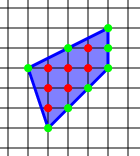Example of a polygon inside a lattice. The red vertices are the lattice points and the green verticles are the boundary points of our polygon.

Since the number of lattice points $i + b$ of our (in our case, this is the rhombus of base and height $n$) is what we are interested in here, we may manipulate picks formula to look like:

Let’s verify this equation when $n=1$. Our rhombus with base and height 1 indeed satisfies Pick’s theoremVerify that $i = 1$, $b = 4$ and $A = 2$ in this case. .

As we rescale the our rhombus by $n$, area gets rescaled by a factor of $n^2$ and the boundary by a linear factor of $n$ (an easy way to see this is to ‘unwind’ the boundary into a line). Therefore, our counting function $G_{\ell^1}$ is given by the following:

and substituing our findings from the $n=1$ case, this gives us the full solution to our counting problem

The takeaway here is that the “round counting” Gauss circle problem is a challenging one, while the “pointy counting” problem, which is to count the number of lattice points contained in and on a n-dilate of a polygon, is fairly straightforward one in comparisonFor the curious, what we just did associating the $n$-dilate of a 2-dimensional polytope with a polynomial has an analogue in d-dimensions, given by the theory of Ehrhart polynomials. .

## Growth of Groups

### Cayley Graph

In the previous, we swapped the metric associated with the $n$-ball from the $\ell^2$-norm to the $\ell^1$-norm, and obtained a whole new counting problem.

We can also swap the lattice $\mathbb{Z}^2$ for a Cayley graph of group!

A Cayley graph $\Gamma(G,S)$ is constructed from a group $G$ by choosing a generating set $S$.

1. Each element $g \in G$ is assigned a vertex.
2. Any $s \in S$ is assigned different directed edge identification (often represented by an arrow).
3. For any $g \in G$, $s \in S$, the vertices corresponding to $g$ and $gs$ are joined by the directed edge $s$. Going the opposite direction from the edge $s$ corresponds to right multiplication by $s^{-1}$.

Let’s look at examples: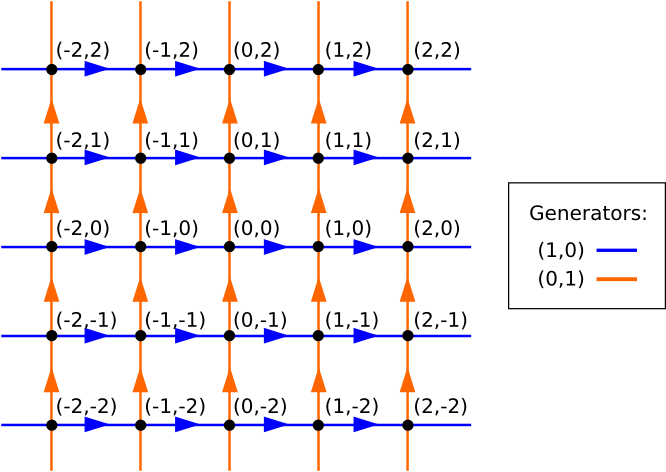A Cayley graph for $\mathbb{Z}^2$.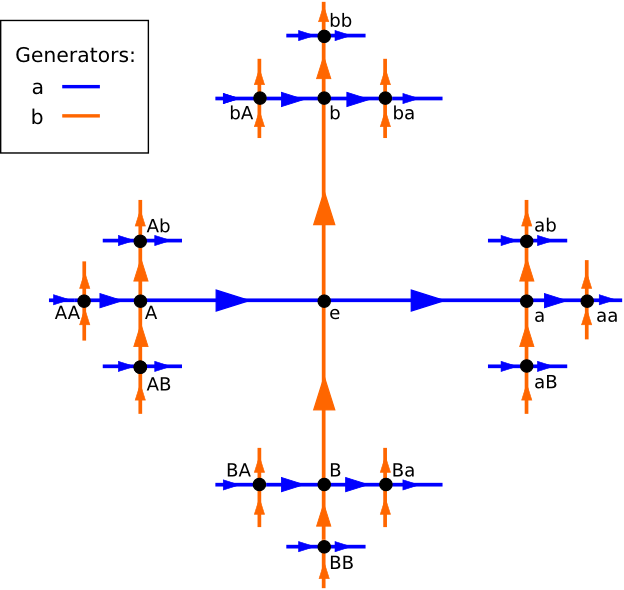A Cayley graph for the free group on two elements, where $a^{-1}$ is denoted by $A$ and $b^{-1}$ by $B$.

Cayley graphs encode all the information contained in a group, because it tells us about all the elements in the group, and how to multiply them together. To figure out what $g_1 g_2$ multiplies to in the group $G$ with generating set $S = {s_1,\dots,s_n}$, we start at $g_1$ in the graph, then follow the path given by the decomposition of $g_2$ into its generators $g_2 = s_{j_1} \dots s_{j_k}$.

A Cayley graph is also equipped with a word metric, denoted $\mid \cdot\mid_S$ by assigning the length $1$ to each edgeThe subscript of $S$ is in the notation to emphasize how the distance is totally dependent on the chosen generating set $S$, which are precisely the edges in the Cayley graph. .

In our Cayley graph of $\mathbb{Z}^2$ with standard generators ${(0,1),(1,0)}$:

• the word-metric ball of radius 0 contains only the point $(0,0)$,
• the ball of radius 1 contains every point that is at most an edge away from the origin, ${(0,0),(1,0),(-1,0),(1,0),(-1,0)}$,
• the ball of radius 2 contains the points that are at most two edges away from the origin,
• and so on.

We can now remark that the ball of radius $n$ defined by the word metric is a rhombus of base and height $n$. Thus, this this word metric ball definition on $\mathbb{Z}^2$ exactly coincides with the $\ell^1$-norm on $\mathbb{Z}^2$!

### Growth function

The growth function of a group $G$ with a generating set $S$ is the number of vertices of the Cayley graph $\Gamma(G,S)$ contained in the ball $B_{\mid \cdot \mid}(n)$ of radius $n$.

If we denote the growth function by $f(n)$$G(n)$ being poor notation here because our group is $G$ , then the growth function is precisely

Punchline: because the Cayley graph of $\mathbb{Z}^2$ is precisely its lattice representation, and because the $\ell^1$-norm on $\mathbb{Z}^2$ is exactly the word metric under standard generators, the growth of $\mathbb{Z}^2$ under standard generators is just the $\ell^1$ counting function!

## Types of Growth: How Fast? How Regular?

There are two crucial and orthogonal properties to the classification of growth in groups.

The first one, the growth rate, is a familiar concept from calculus. The second one, the rationality of the growth, uses generating functions.

### Growth Rate and the Classification Problem

The first one is a familiar one from calculus. The growth rate of a group quantifies how fast a group grows as $n \to \infty$.

The growth rate of the free group on two elements we saw earlier is exponential as its Cayley graphs will always be a tree with many splittings, independently of the choice of generating setI’m being handwavy here because I can’t think of an actual formal argument right now. Let me know if you know one! .

On the other hand, the growth function of $\mathbb{Z}^2$ with standard generating set was found to be a polynomial of degree 2 using Pick’s theorem, and in fact, it can be proven using the Pick’s theorem that the growth function of $\mathbb{Z}^2$ is always a polynomial of degree 2 regardless of generating set. We say that the growth of $\mathbb{Z}^2$ is polynomial.

Notice that we say “how fast a group grows”, and do not specify the generating set. This is because changing the generating set induces a quasi-isometry, which for our purposes is a transformation that does not distort distance by more than an affine transformation. Because an affine transformation does not change the large-scale asymptotitc behavior of a function (exponential, polynomial), we can truly talk about the growth of a group.

One might wonder if exponential growth and polynomial growth stems from some underlying geometry of groups, such that all tree-like groups (known as hyperbolic groups) grow exponentially, and all abelian groups grow polynomially. The answer is affirmative. In fact, we have an even stronger answer.

A 1981 theorem of Gromov states that the virtually nilpotent group are exactly the groups with growth in the polynomial range.

A group $G$ is nilpotent if there is an $n$ such that for arbitrary elements $g_i$ of $G$, the iterated commutator $[\dots[[g_1, g_2],g_3],g_4]\dots g_n] =1$Recall that the commutator of $g_1, g_2$ is denoted by $[g_1,g_2] = g_1 g_2 g_1^{-1} g_2^{-1}$, and can be thought of as measuring the failure to commute. Our object $[\dots[[g_1, g_2],g_3],g_4]\dots g_n]$ denotes iterated commutators (commutators of commutators of…commutators)! . Abelian groups are nilpotent groups of step 1, as their commutators are the identity after one iteration. Non-examples of nilpotent groups would be free groups. In some sense, a nilpotent group is a group that “finitely many steps away from being abelian”.

A great example of a nilpotent group is the Heisenberg group given by the presentation

It is a nilpotent group of step $2$, because while its commutator $[a,b]$ does not vanish, it commutes with everythingby definition of being central . Thus, the commutator of the commutator must vanish.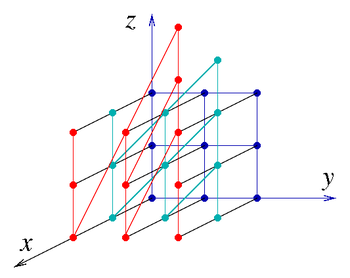This pretty terrible Cayley graph of the Heisenberg group (the colors do not represent the generators) is trying to show that the Heisenberg group has the characteristic "twisty" geometry of nilpotent group. In contrast, its counterpart $\mathbb{Z}^3$ is very "flat" in its geometry.

A group is virtually nilpotent if it has a nilpotent subgroup of finite index. Since we care about the asymptotic behaviour of growth, we focus on infinite groups as otherwise the growth is simply constant as $n \to \infty$. Thus, assuming the a group is infinite, a group is virtually nilpotent if its nilpotency is “significant enough”.

A group has growth in the polynomial range if its growth function $f(n)$ satisfies for some degree $d$ the relation

note that the limit is allowed not to exists, which happens when the growth function is a polynomial with periodic coefficients For example if $f(n) = n$ for $n$ odd, and $f(n) = 2n$ for $n$ even. .

Gromov’s theorem was remarkable in that it completely classified the growth rate of an enourmous class of groupsSome trivia: In 2007, Bruce Kleiner gave a new and simplified proof. Three years later in 2010, Yehuda Shalom and Terence Tao gave a quantitative version of Gromov’s theorem. . While the classification of finite groups has been done in the last century, the classification of infinite groups is still in progress, and notoriously difficult. There are uncountably many infinite groups, and Dehn’s isomorphism problem (given two groups, figure out whether they are isomorphic) is algorithmically undecidable.

Justly, Gromov’s breakthrough induced a spur of activity and interest in the growth of groups, which continues to this day.

### Rationality and the Word Problem

Consider the Fibonnaci-style sequence of rational numbers $a_n$, which satisfies the famous recursion

We can associate to its generating function given by the formal power series

From the recursion, we find that

and factoring the $\mathbb{A}(x)$ gives us

Because the generating function of our sequence $a_n$ can be written as a ratio of two polynomials, we say that $a_n$ has rational growth.

It is a well-known fact that a sequence is rational if and only if it satisfies a Fibonnaci-style recursion of integer coefficients and finite depth $d$

The rationality of a sequence has nothing to do with its growth rate. Indeed, the sequence

has rational growth, while the generating function based on the sequence of digits of $\pi$,

which is bounded away from the rational sequence

cannot be rational, otherwise for some polynomial $p,q \in \mathbb{Q}$

Thus, two sequences having the same growth rate do not necessarily share this rationality property.

We can easily apply this framework to growth functions of groups by viewing $f(n)$ as the sequence $\beta_n = f(n)$We use $\beta$ for ball count, viewing $\beta_n$ as the number of points inside the ball of radius $n$ under the word metric of the Cayley graph. . Just as the rationality of a sequence can be destroyed by adding in a bounded sequence, changing the generating set, which induces an essentially affine change in our growth function, can destroy rationality. While growth rate is independent of choice of generators, rationality does depend on the choice of generators.

This begs the question which groups have rational growth, and in what generators?

Or perhaps, why do we care at all?

Essentially, rationality allows us to construct the growth of a group using very little initial information, and leads to a halting condition for the word problem.

The growth of a group $\beta_n$ gives us the number of words of length $\leq n$, and so a simple substraction $\sigma_n = \beta_n - \beta_{n-1}$We use $\sigma$ for sphere count. $\sigma_n$ denotes the discrete sphere of radius $n$. gives the number of group elements which are exactly of length $n$.

Then, for a spelling of length $n$, since we know about many words (equivalence class of spellings) there are, we can compare every spelling with one another. Suppose there are $\sigma_n$ such equivalence classes of spellings. Inserting relations for two spellings of the same equivalence class, these two spellings will end up equal after a finite amount of steps. Otherwise, if the two spellings happen to be in different equivalence classes, then the process of determining they are equal by using the relations will not terminate. Nonetheless, the overall process will terminate because eventually, $\sigma_n$ spellings will reveal themselves to be the same, and this is when we decide to terminate the processThanks to Felix Bauckholt for helping me clarify this! .

In 1980s, it was shown by Cannon, Thurston, Gromov and Benson that if the group is flat (virtually abelian) or hyperbolic, then the growth is rational under all generating sets.

## The Curious Nilpotent Regime

Recall that we introduces three geometric regimes: the hyperbolic regime, which captures the tree-likeness of free groups, the flat regime, which captures abelian groups, and the nilpotent regime, which a flat regine with some twist.

Curiously, the nilpotent regime shares with abelian groups a polynomial growth rate, but is somewhere in between rational and transcendental when it comes to growth structure.

A concrete example is the discrete Heisenberg group of dimension 5, denoted

not to be confused with the Heisenberg group of dimension 3Confusingly also called the Heisenberg group.

which we have also seen presented as

$H_5(\mathbb{Z})$ has transcendental growth in its standard generating set, but rational growth in a generating set called cubical.

In contrast, the Heisenberg group of dimension 3 has rational growth in all of its generators, a fact that has only been proven very recently by Moon Duchin and Michael Shapiro.

Currently, little is known about these nilpotent groups and they are according to Duchin a very underappreciated area of study ;).

My supervisor Moon Duchin has excellent material on the topic. I highly recommend the following:

1. AMS Notices article
2. Lecture series given at the Institute of Advanced Studies.

Groups of Intermediate Growth: an Introduction for Beginners by Grigorchuk and Pak settles the question whether there are groups with growth rate faster than polynomial but slower than exponential. Also a great read I should read!

There is this great REU paper by George Hyun on Hyperbolicity and the Word Problem which I’m looking forward to reading!

For those who have a good amount of background on this topic, I am also trying to read A Short Course in Geometric Group Theory by D. Neumann and Michael Shapiro. Although written in 1996, I am finding it to be a treasure trove of concepts for the literature on growth today. I am far from being able to catch every concept that is referred to, and would love to acquire a reading buddy to discuss these concepts with me :).

As always, feel free to email me if you have any questions, or want to start a discussion on the topic.

Many thanks for Felix Bauckholt for proofreading this and giving insightful feedback!

You may wonder if there is growth in the intermediate range, i.e. between polynomial and exponential. The answer is affirmative.

Define a function $f(n)$ to be superpolynomial if

This captures the behaviour that “$f(n) \geq n^\alpha, \alpha \to \infty \text{ as } n \to \infty$”.

Define a function $f(n)$ to be subexponential if

This captures the behaviour that “$f(n) \leq e^{\alpha n}, \alpha \to 0 \text{ as } n \to \infty$”.

A function has intermediate growth if it has both superpolynomial and subexponential growth.

An example of this is the function $n^{\log log n}$.

In their paper Groups of Intermediate Growth: an Introduction for Beginners Rostislav Grigorchuk and Igor Pak construct a group of intermediate growth by looking at the group of automorphisms acting on a tree. I highly recommend this paper, a gem written at an accessible level.Honestly I should sit down and read it in full myself.

It is conjectured by Pak and Grigorchuk that there are no finitely presented groups with intermediate growth – meaning this whether a finitely presented group either has polynomial or exponential growth is an open problem!I don’t know if I’m up to date though!

Introducing Growth of Groups - March 14, 2017 - Hang Lu Su